Representing random functions with the Karhunen-Lòeve expansion

### The uncertainty quantification series continues

Did you end up at this post by chance? Then it might be a good idea to start from the beginning, to learn what uncertainty quantification is in the first place.

Once you’ve made it back this far, you’ll have learned about polynomial chaos. Quite simple really – just writing the random variable as a sum of polynomials, with a nicer random variable as the argument.

### More variables

But what if we have more variables than just one? Specifically, what to do if the variables are statistically dependent? This is quite common, especially if the variables are related to the same quantity. Like the resistances of two coils wound from the same spool of wire, or the characteristics of two nearby welding seams.

And it can go further than this. What if we don’t simply have several variables – what if we have infinitely many? Because that’s what we’ve got when we have a random function. (A more exact term would probably be random field, but a function‘s easier to understand.) After all, the value of the function at each and any point is a random variable. And there are infinitely many points.

An interesting random function might be for instance the magnetic permeability, as that’s known to vary quite a bit. The quality and characteristics of the winding resin could be another. The impregnation process is not a perfectly exact science, and even after that all the mechanical stresses might generate cracks and faults. In mechanics, welding seams can exhibit quite a bit of variability. Likewise, any thin shell-like structure might have some dents, which can radically affect its loadability.

So, how do we deal with more than one random variable?

### Independent or not

Things are simple if the random variables are statistically independent. Meaning they are not correlated, nor related or dependent in any other way either. In that case, we can simply write separate polynomial expansions for each of them, and call that a day.

Obviously, this approach cannot be applied to random functions. They are essentially infinite-dimensional random variables after all. Writing an infinite number of expansions doesn’t sound very practical, does it?

Furthermore, random functions don’t fit into the independency assumption thingy at all. Think of it. Let’s say our permeability is a random function. Now let’s look at the permeability at two point, one tenth of a millimeter apart. Think they are ever going to be totally diffent from each other? Not a change (pun intended), unless the material has a crack or something.

Indeed, the values of a random function are pretty much always at least somewhat dependent, at least locally. In simpler terms, the value of the function at any point, influences its value at the nearby points.

#### Dependent how?

Like we learned earlier, determining the complete probability distribution of a random function is quite complex indeed. Instead, we usually opt for something simpler.

Indeed, we look at two things. One is the marginal distribution of the function – how its value at any given point behaves, regardless of what happens anywhere else.

The second is its covariance function, or covariance kernel. As its name suggests, the covariance function measures the covariance between the function values at two different (or same) points.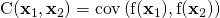.

The higher the covariance, the more correlated the two points are with each other.

The covariance function is typically also the first step we take when analysing random functions. We’ll soon see how.

But first – the first things.

### Expansions for functions

So, we have a random function. To really stress that it’s dependent on the position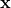, let’s write it as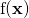. Furthermore, it’s also dependent on what happens by chance, and that kind of dependency is usually denoted by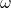or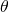. Sinceis a common symbol for angular frequency for us electrical engineers, I’ll use.

Thus, we writeas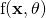. Simply to signify that it’s dependent on both the position and chance.

This double-dependency is difficult to handle.

To solve this problem, we writeas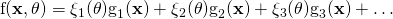.

Here, all the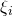are simple random variables – so no dependency on position. By contrast,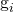are deterministic functions of position – no randomness here.

And we know how to handle both.

The position-dependent functions are simply, well, functions. Everybody knows how to handle a function. For the record, they are often called modes.

For the random variables, on the other hand, we can use for…guess what? The polynomial chaos approach I’ve been blabbering about. There’s no special name for the variables as far as I’m aware.

And the act of writingas a sum of something else – that’s called an expansion.

#### Getting the expansion

So, how to determine the modes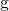and the variables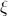? For that purpose, something called Karhunen-Loève theorem (KL) is often used.

“Simply” put, the we first solve some eigenvalues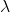and eigenfunctions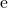satisfying the integral equation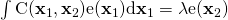.

To achieve that, we can first discretize the problem with finite element analysis, and then solve a matrix eigenvalue problem. Heavy for your computer, but doable. In practice, we’ll often need several eigenfunctions rather than simply one.

Once we have the solution, we get the desired functions for the expansionby simply setting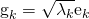.

That’s simply the magic of Karhunen-Lòeve at work.

#### Getting the random variables

We also know that the random variableswill be uncorrelated, and each of them will have an unit variance. That’s for sure, again thanks to the KL magic.

In the simplest case, they will all be normally distributed and statistically independent. This happens when our original functionis Gaussian.

In more complex cases, things get a little shady.

Allwill still be assumed statistically independent. The reason for this is simple – nobody knows what else to do. This is a limitation, yes, but usually not a bad one. The variables will in any case be uncorrelated, so in practice assuming then independent rarely does bad damage.

So, the remaining thing is to simply determine the distribution of each. For this, an iterative procedure is usually performed. Specifically, we update our estimates for each variable, until the marginal distributions of the expandedmatch the desired value. Once they do, we are as close as we can get to the true behaviour.

Finally, when we know the distribution, we write eachwith the polynomial chaos expansion. Exactly like we learned to do last time.

### Conclusion

Today, we learned how to expand a random function with the Karhunen-Lòeve expansion. The function is written as a sum of deterministic functions (of position x), multiplied by scalar-valued random variables. We also learned how to determine these special functions and variables.

Next time, we shall learn how all this mathematical awfulness can be used in practical applications.

Until then!

-Antti

Need help with electric motor design or design software? Let's get in touch - satisfaction guaranteed!What is Karhunen-Lòeve Expansion?